﻿ BioLogica .Catalogue, Instructions, Videos
Biomedicals
Product Information
Summer School
Grapher and Unit Converter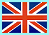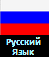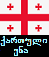# Seminars

1) Construction of calibration curves

During the construction of calibration curves, student learns: dilution measuring and graphing. Student begins to understand the meaning of the standard solutions and the calibration curve, which dassically (see Fig. 1) has three zones:

1. Not a sensitive area - is rarely present in real experiments
2. Directly proportional to the area (first-order reaction)
3. Zone pinning (zero order reaction)

Zone 1 is when there is not enough sensitivity for a certain compound connection and with the increase of concentration of the bond the optical density is either not increasing, or increasing disproportionately. Zone 2 is a directly proportional relationship, expressed mathematically by y = ax + b. This area, called the working area 2 - first-order reaction . That is, the student is explained that both the optical density and concentration of the various compounds should not exceed the performance zone. The figure shows that during the experiment OD values may range from 0.6 to 1.7 index absorption and from 1.1 to 2.15 or the amount of concentration of the test compound. All values obtained above or below for the calculations are incorrect. In the case of the high rate than in zone 2 , the values go in zone 3 of the curve. In this area, as in zone 1 (due to the fact that it is not directly proportional to ) is not correctly calculate values.

Zone -1 Zone 1 is the area of the curve, when there is no sensitivity or the method or apparatus. With increasing concentration of the compound or the optical density does not increase, or disproportionately increasing, until reaching the zone 2.

Zone -2 Zone 2 , the curve is proportional relationship , expressed mathematically y = ax + b. The intersection of the curve and the tangent - workspace - first-order reaction. Optical density and concentration of various compounds should not go beyond the parameters, zone -2 . The figure shows that the OD values can be from 0.6 to 1.7 index absorption, and from 1.1 to 2.15, the concentration of (or measurable amounts of compound) .All values obtained above or below for the calculations are incorrect.

Zone -3 If the figures (analytic) Higher performance than in zone 2, the values are in zone 3 . In this area , as in zone 1 (due to the fact that it is not directly proportional to) is not correctly calculate values . In the case of high rate, the sample is diluted to as long as optical density will not appear in zone 2.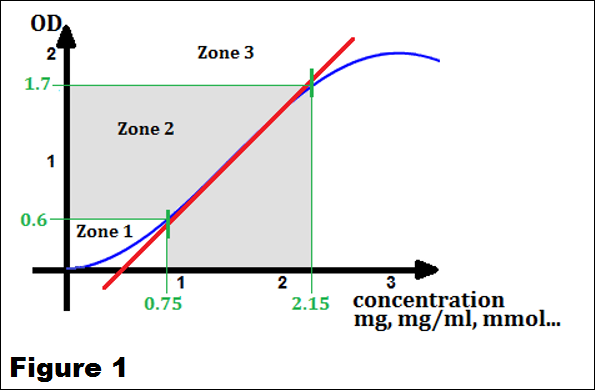2) Determination of the concentration of biologically active compounds

Practical methods include determining the concentration of glucose and protein in a biological extract (in this case milk hydrolyzate and hydrolyzate concentrate (condensed milk). Values of protein and glucose in the hydrolyzate of milk should be in the second zone. Given that this area is directly proportional dependence can perform calculations using the following methods : Graphical determination (applies when the calibration curve is stable and there is no danger of her shift, and when saving reagent) The protein solution or a glucose solution is added to a solution of the chromogen . OD value obtained graphically transform into a quantitative indicator. Example, see Figure 1. If the OD of the title compound was 0.6 then it will be equal to the concentration of 0.75 mg . Measurements with the standard solution (used if there is danger of a shift of the curve or angle change) . According to the standard solution, if the OD is in zone 2, the calculation is carried out , the chemical - proportion: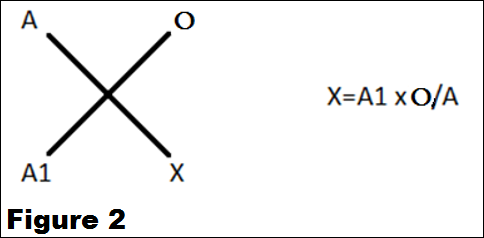where A - the optical index of the standard solution , O - concentration of the respective standard A1 - the optical index of the desired solution X - concentration of the desired solution. Example, see Fig. 1. Measured OD standard (eg 2.15mg/ml ) and the test solutions. Absorbance value of the standard solution should correspond to 1,7 OD ( see figure 1 ) . Assume that OD- 0.6 test solution then, according to the proportion of X = 0.6 X2.15/1.7. The resulting value of X = 0.75 - coincides with the graphical indicator.Calculation using factor F ( applies to cases where the reagent is very stable ) . Calculation using factor is a simplified method of calculation , standard so as the ultimate formula calculated with the standard is X = A1 * B / A where B / A- known parameters of the standard solution , and dividing one by the other , we obtain a factor .-F. Example, see Fig. 1. Measured OD standard solution such .2.15mg/ml . Absorbance value of the standard solution should correspond to 1.7 OD (Fig. 1). calculated factor F = 2.15/1.7, F = 1.26. Assume that OP- test solution is 0.6. Then, according to the proportion of X = 0.6 * F ie X = 0.6 * 1.26 i.e. X = 0.75. The resulting value of 0.75 corresponds to a graphical indicator, and calculated with the standard. All three options counting legitimate if the calculated figures are in zone 2 - see Figure 1 . Indicators concentrated condensed whey should be in zone 3 . Therefore, this sample diluted twice with saline , and measurement is made again. Due to the fact that the sample is diluted 2-fold after the calculation value obtained is multiplied by the number of dilutions. The calculations can be carried out by any of the above three methods.

3) Enzymology

3.1) Determination of the enzyme- substrate dependence and constant Michaelis-Menthen (Km)

A student at this stage of development, can measure glucose, which is the product of hydrolysis of sucrose , and protein that is, the enzyme catalyzing the reaction . Accordingly, we introduce the concept of the enzyme. In this case, invertase , wherein the substrate is sucrose . But the student is not yet ready to discuss about the activity as the main problem of the relationship of the enzyme [ E ] and substrate [S] is not yet clear . Basic postulate is the relationship [S] = 10km . This before we measure the activity , the student must determine Km to calculate the exact concentration of substrate , time, temperature , i.e. must simulate method determining the activity and then measured. Take the excess concentration of sucrose , add the enzyme and for a minimum period of time (time to achieve maximum detection product) , glucose is measured as a product . In the subsequent tubes , diluted sucrose (concentration of the enzyme , and the time does not change ) until it starts to decrease enzyme activity . Reaching invertase activity indicators reduction due to reduction of the substrate concentration is plotted the velocity of the reaction ( activity or concentration of the substrate). The resulting dependence depicts equation Michaelis-Menthen (Km).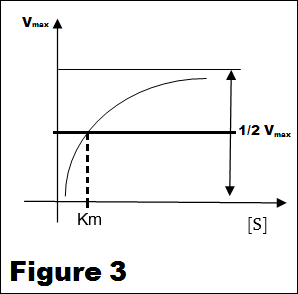The Michaelis-Menten equation arises from the general equation for an enzymatic reaction: E + S ↔ ES ↔ E + P, where E is the enzyme, S is the substrate, ES is the enzyme-substrate complex, and P is the product. Thus, the enzyme combines with the substrate in order to form the ES complex, which in turn converts to product while preserving the enzyme.

Km is the Michaelis-Menten constant which shows the concentration of the substrate when the reaction velocity is equal to one half of the maximal velocity for the reaction. It can also be thought of as a measure of how well a substrate complexes with a given enzyme, otherwise known as its binding affinity. An equation with a low Km value indicates a large binding affinity, as the reaction will approach Vmax more rapidly. An equation with a high Km indicates that the enzyme does not bind as efficiently with the substrate, and Vmax will only be reached if the substrate concentration is high enough to saturate the enzyme.
Taking the reciprocal of both side of the Michaelis-Menten equation gives: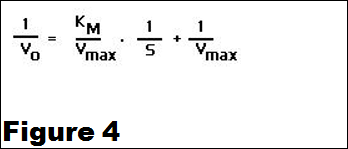To determined the values of KM and Vmax. The double-reciprocal of Michaels-Menten equation could be used. For this, the Michaelis-Menthen equation can be transformed into the Lineweaver-Burk equation.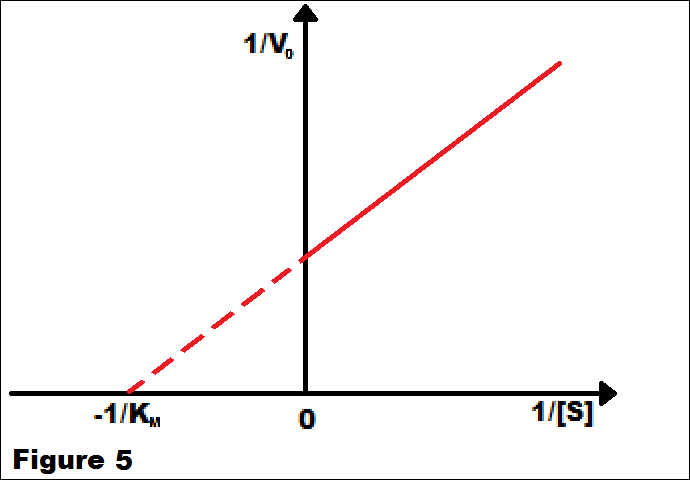Lineweaver-Burk Plot

A graph of the double-reciprocal equation is also called a Lineweaver-Burk, 1/Vo vs 1/[S]. The y-intercept is 1/Vmax; the x-intercept is -1/KM; and the slope is KM/Vmax. Lineweaver-Burk graphs are particularly useful for analyzing how enzyme kinematics change in the presence of inhibitors, competitive, non-competitive, or a mixture of the two. Graphically calculated value Km. From the condition that the substrate concentration [S] must be equal to 10Km, i.e. [S] = 10 * Km. simulate the experience to determine the enzymatic activity of invertase.

3.2) Determination of the enzymatic activity of invertase

The chemistry: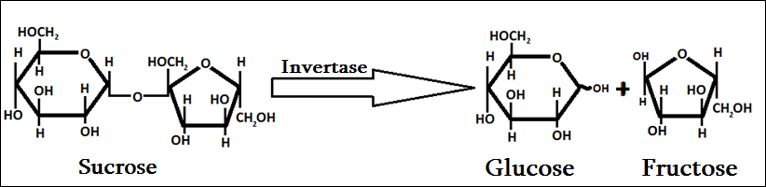The proposed sets of Km obtained experimentally should be equal to 20 (± 10%). Accordingly, the substrate concentration , to determine the activity will be 200mg/ml . Invertase activity is determined with the same enzyme concentration , which was used in the method of Michaelis- Menten . Take the calculated substrate concentration of 200 mg / ml , and the time for determining the activity is chosen so that the resulting product ( glucose) was in zone 2 (in this case 10 minutes) maximum value. Activity per ml of solution computed by the formula: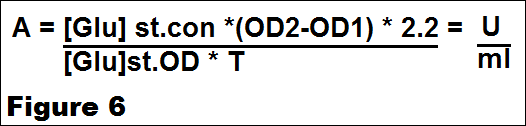OD - optical density of the sample , T - time of the reaction ( 100) - the factor for converting (1) ml. [Gluk.st] - concentration of a standard solution of glucose , [Gluk.std.OD] - the absorbance of the standard solution of glucose. Measured protein concentration . the ratio of activity (ml ) , and the protein concentration gives a specific ( relative ) activity A (specific ) = A U / ml / Mg / ML = A U / Mg.

3.3) Training method, protein precipitation and salting

Comparison of total and specific activities
Most often in enzymology , the first stages of purification of enzymes used salting or precipitation . Most often it is caused by problems with fractionation and cleaning up . The purpose of laboratory workshop is to introduce the methods of salting out and precipitation. Shows a decrease in the total activity associated with the loss of the enzyme , and the increase in specific activity associated with cleaning out .

3.4) Hydrolysis of sucrose

One of the common tasks in biotechnology, is the hydrolysis of a substrate to a final product. In this case, the question can be formulated as follows: remove the sucrose from the solution. From the standpoint of industrial problems , the matter may stand as follows: get fructose, glucose or glucose- fructose syrup ( inversion , sugar) problems encountered in the field of analytical - hold , Quantitative analysis of sucrose. All of the above problems associated with the hydrolysis of sucrose. The problem may be inhibition of the enzyme , its inactivation and so .. The very posing of this question involves the optimal selection of optimal conditions for the hydrolysis , i.e. determining the time , pH and temperature hydrolysis , and determining the concentration of enzyme and substrate , and most importantly determine the maximum depth of hydrolysis , ie the conditions under which the transformation occurs maximum sucrose to invert sugar. The problem can be stated as follows : the maximum transfer to the hydrolysis products of sucrose , minimum flowrate to achieve maximum enzyme transforming the hydrolysis products of sucrose , as well as to the minimum time to achieve hydrolysis of sucrose. In this experiment we accept a priori that parameters such as temperature pH , enzyme concentration , and substrate are optimal . Vareabelnym parameters , subject matter of time , ie the time in which the chosen concentration enzyme and substrate at pH -4,5, temperature 55 °, the maximum hydrolysis of sucrose hold (in this case, .....? hours).

4) Chromatography

Thin Layer Chromatography (TLC)

TLC is a simple, quick, and inexpensive procedure that gives the chemist a quick answer as to how many components are in a mixture. TLC is also used to support the identity of a compound in a mixture when the Rf of a compound is compared with the Rf of a known compound (preferably both run on the same TLC plate). A TLC plate is a sheet of glass, metal, or plastic which is coated with a thin layer of a solid adsorbent (usually silica or alumina). A small amount of the mixture to be analyzed is spotted near the bottom of this plate. The TLC plate is then placed in a shallow pool of a solvent in a developing chamber so that only the very bottom of the plate is in the liquid. This liquid, or the eluent, is the mobile phase, and it slowly rises up the TLC plate by capillary action. As the solvent moves past the spot that was applied, an equilibrium is established for each component of the mixture between the molecules of that component which are adsorbed on the solid and the molecules which are in solution. In principle, the components will differ in solubility and in the strength of their adsorption to the adsorbent and some components will be carried farther up the plate than others. When the solvent has reached the top of the plate, the plate is removed from the developing chamber, dried, and the separated components of the mixture are visualized. If the compounds are colored, visualization is straightforward. Usually the compounds are not colored, so a UV lamp is used to visualize the plates. (The plate itself contains a fluorescent dye which glows everywhere except where an organic compound is on the plate.)

The Rf value

The retention factor, or Rf, is defined as the distance travelled by the compound divided by the distance travelled by the solvent.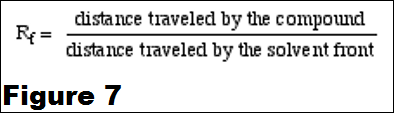For example, if a compound travels 2.1 cm and the solvent front travels 2.8 cm, the Rf is 0.75: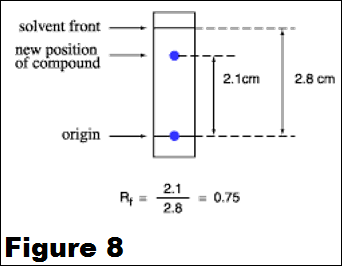The Rf for a compound is a constant from one experiment to the next only if the chromatography conditions below are also constant:
•        solvent system
•        amount of material spotted
•        temperature
Since these factors are difficult to keep constant from experiment to experiment, relative Rf values are generally considered. "Relative Rf" means that the values are reported relative to a standard, or it means that you compare the Rf values of compounds run on the same plate at the same time. The larger an Rf of a compound, the larger the distance it travels on the TLC plate. When comparing two different compounds run under identical chromatography conditions, the compound with the larger Rf is less polar because it interacts less strongly with the polar adsorbent on the TLC plate. Conversely, if you know the structures of the compounds in a mixture, you can predict that a compound of low polarity will have a larger Rf value than a polar compound run on the same plate. The Rf can provide corroborative evidence as to the identity of a compound. If the identity of a compound is suspected but not yet proven, an authentic sample of the compound, or standard, is spotted and run on a TLC plate side by side (or on top of each other) with the compound in question. If two substances have the same Rf value, they are likely (but not necessarily) the same compound. If they have different Rf values, they are definitely different compounds. Note that this identity check must be performed on a single plate, because it is difficult to duplicate all the factors which influence Rf exactly from experiment to experiment.

Troubleshooting TLC

All of the above (including the procedure page) might sound like TLC is quite an easy procedure. But what about the first time you run a TLC, and see spots everywhere and blurred, streaked spots? As with any technique, with practice you get better. Examples of common problems encountered in TLC:
•        The compound runs as a streak rather than a spot: The sample was overloaded. Run the TLC again after diluting your sample. Or, your sample might just contain many components, creating many spots which run together and appear as a streak. Perhaps, the experiment did not go as well as expected.
•        The sample runs as a smear or a upward crescent: Compounds which possess strongly acidic or basic groups (amines or carboxylic acids) sometimes show up on a TLC plate with this behavior. Add a few drops of ammonium hydroxide (amines) or acetic acid (carboxylic acids) to the eluting solvent to obtain clearer plates.
•        The sample runs as a downward crescent: Likely, the adsorbent was disturbed during the spotting, causing the crescent shape.
•        The plate solvent front runs crookedly: Either the adsorbent has flaked off the sides of the plate or the sides of the plate are touching the sides of the container (or the paper used to saturate the container) as the plate develops. Crooked plates make it harder to measure Rf values accurately.
•        Many random spots are seen on the plate: Make sure that you do not accidentally drop any organic compound on the plate. If get a TLC plate and leave it laying on your workbench as you do the experiment, you might drop or splash an organic compound on the plate.
•        You see a blur of blue spots on the plate as it develops: Perhaps you used an ink pen instead of a pencil to mark the origin?
•        No spots are seen on the plate: You might not have spotted enough compound, perhaps because the solution of the compound is too dilute. Try concentrating the solution, or spot it several times in one place, allowing the solvent to dry between applications. Some compounds do not show up under UV light; try another method of visualizing the plate (such as staining or exposing to iodine vapor). Or, perhaps you do not have any compound because your experiment did not go as well as planned. If the solvent level in the developing jar is deeper than the origin (spotting line) of the TLC plate, the solvent will dissolve the compounds into the solvent reservoir instead of allowing them to move up the plate by capillary action. Thus, you will not see spots after the plate is developed. These photos show how the yellow compound is running into the solvent when lifted from the developing jar.

Gel filtration chromatography

Gel filtration chromatography is a separation based on size. It is also called molecular exclusion or gel permeation chromatography. In gel filtration chromatography, the stationary phase consists of porous beads with a well-defined range of pore sizes. The stationary phase for gel filtration is said to have a fractionation range, meaning that molecules within that molecular weight range can be separated. Proteins that are small enough can fit inside all the pores in the beads and are said to be included. These small proteins have access to the mobile phase inside the beads as well as the mobile phase between beads and elute last in a gel filtration separation. Proteins that are too large to fit inside any of the pores are said to be excluded. They have access only to the mobile phase between the beads and, therefore, elute first. Proteins of intermediate size are partially included - meaning they can fit inside some but not all of the pores in the beads. These proteins will then elute between the large ("excluded") and small ("totally included") proteins. Consider the separation of a mixture of glutamate dehydrogenase (molecular weight 290,000), lactate dehydrogenase (molecular weight 140,000), serum albumin (MW 67,000), ovalbumin (MW 43,000), and cytochrome c (MW 12,400) on a gel filtration column packed with Bio-Gel P-150 (fractionation range 15,000 - 150,000). When the protein mixture is applied to the column, glutamate dehydrogenase would elute first because it is above the upper fractionation limit. Therefore it is totally excluded from the inside of the porous stationary phase and would elute with the void volume (V0). Cytochrome c is below the lower fractionation limit and would be completely included, eluting last. The other proteins would be partially included and elute in order of decreasing molecular weight. These separations can be described by this equation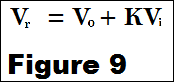where Vr is the retention volume of the protein, V0 is the volume of mobile phase between the beads of the stationary phase inside the column (sometimes called the void volume), Vi is the volume of mobile phase inside the porous beads (also called the included volume) and K is the partition coefficient (the extent to which the protein can penetrate the pores in the stationary phase, with values ranging between 0 and 1). In the mixture of proteins listed above, the partition coefficient (K) for glutamate dehydrogenase would be 0 (totally excluded), K = 1 for cytochrome c (totally included) and K would be between 0 and 1 for the other proteins, which are within the fractionation range for the column. In practice, gel filtration can be used to separate proteins by molecular weight at any point in a purification of a protein. It can also be used for buffer exchange - a protein dissolved in a sodium acetate buffer, pH 4.8, can be applied to a gel filtration column that has been equilibrated with tris buffer, pH 8.0. Using the tris buffer, pH 8.0, as the mobile phase, the protein moves into the tris mobile phase as it travels down the column, while the much smaller sodium acetate buffer molecules are totally included in the porous beads and travels much more slowly than the protein.

Ion-Exchange Chromatography

Ion exchange chromatography is the reversible adsorption of charged molecules to immobilized ion groups on a matrix of an opposite charge. Separation can be selectively achieved by adsorption and release of samples from the matrix. Ion exchange starts with the equilibration of the exchanger using pH, and ionic strength. During equilibration the exchangable groups are associated with counter ions. Once equilibrium is reached and the sample added the molecules undergo addition and adsorption with an appropriate charge displace the counter ions and bind reversibly to the matrix. The unbound materials will pass through the column with the void volume. In the third stage, substances are removed from the column by increasing the ionic strength of the eluting buffer. Ion-exchange media is an insoluble matrix with charge groups covalently attached. Negatively charged exchangers bind positively charged ions (cations). Exchangers bind one type of cation but, when presented with a second type of cation, it may displace, and/or exchange with, the first. These resins are called cation-exchangeresins. Anion-exchange resins are positively charged and bind and/or exchange negatively charged ions (anions). Several side-chain groups of the amino acid residues in proteins are ionizable (e.g.lysine or glutamic acid) as are the N-terminal amino and C-terminal carboxylgroups and so proteins are charged molecules. This characteristic can be used to separate different proteins by ion-exchange chromatography.

Two weak exchangers can be used for protein separation are carboxymethylcellulose(CM-cellulose) and diethylaminoethyl-cellulose (DEAE-cellulose). These covalently cross-linked agarose bead ion celluloses have been chemically modified.CM-cellulose has a carboxymethyl functional group -CH2OCH2COOH. At neutral pH the carboxymethyl group is ionized as -CH2OCH2COO¯ so that Cmcellulose is negatively charged, so it is a weak cation exchanger. DEAE-cellulose contains an diethylaminoethyl group. It is positively charged at neutral pH and so DEAE-cellulose is a weak anion exchanger. The Sepharose types are particularly useful for the separation of high molecular weight proteins. In practice, since these matrices are very similar to those used forgel filtration some molecular sieving may accompany the ion-exchange process. This may either enhance or reduce the effectiveness of the fractionation compared to using an ion-exchange cellulose.Two strong exchangers are Q-Sepharose Fast Flow and SP-Sepharose Fast Flow. The charged group of Q-Sepharose is a quarternary amine which carries a nontitratable positive charge. This matrix can be used at alkaline pH values at which the positive charge of the DEAE group would have been titrated. The charged group of S-Sepharose is the sulphonyl group (-SO3¯). The fractionation of proteins by ion-exchange chromatography depends upon differences in the charge of different proteins. The charge of a protein depends upon the number and type of ionizable amino acid side chain groups. Lysine residues, have a positively charged side chain group when ionized, whereas glutamic acid residues are negatively charged when ionized. Each ionizable side chain group has a distinct pKa; that is, the pH at which it is half dissociated. Therefore the overall number of charges on a particular protein at a particular pH will depend on the number and type of ionizable amino acid side chain groups it contains. Since, by definition, different proteins have different amino acid compositions, they will tend to have different charges at a given pH and so can be fractionated on this basis. Each protein has a isoelectric point (pI) where at a certain pH the overall number of negative charges equals the number of positive charges and so it has no net charge. The pI, is the proteins isoionic point. When a protein is at its pI the protein will not bind to the ion-exchange resin. Below this pH the protein will have a net positive charge and will bind to a cation exchanger, and above this pH it will have a net negative charge and bind to an anion exchanger. In principle, either a cation exchanger or an anion exchanger to bind the protein of interest.

In practice proteins are stable and functionally active within a fairly narrow pH range so the choice of ion exchanger is often dictated by the pH stability of the desired protein. If the protein is stable at pH values below its pI, a cation exchanger should be used if it is stable at pH values above its pI, an anion exchanger would be chosen. If a protein is stable over a wide pH range, then either type of media should be tried and the selection depends on the best fractionation.

Ion-exchange media, usually have the ions bound to the charged groups on the resin are called ‘counter ions’. For CM-cellulose the counter ion is usually Na+ and for DEAE-cellulose the counter ion is normally Cl¯. After choice of the appropriate resin, it is mixed with buffer to form a slurry which is poured into a suitable chromatography column. The pH of this starting buffer is crucial since it will determine the charge on the proteins to be separated. The starting buffer pH should be at least one pH unit above or below the pI of the protein to be bound to the resin to ensure adequate binding. However, bear in mind that CM-cellulose and DEAE-cellulose are examples of weak ion exchangers. A weak ion exchanger is one which is ionized over only a limited pH range. Thus DEAE-cellulose begins to lose its charge above pH 9 whilst CM-cellulose begins to lose its charge below about pH 5. The term ‘weak’ does not refer to the strength of binding of ions to the resin nor to the physical strength of the resin itself. With these points in mind then, the effective starting pH range when using DEAE-cellulose or CM-cellulose is only about pH 5 - 9. In addition to correct choice of the pH of the starting buffer, one should take care that its ionic strength is reasonably low since the affinity of proteins for ion-exchange resins decreases as ionic strength increases. Indeed this property is used in one method of eluting the bound proteins.